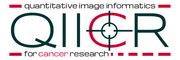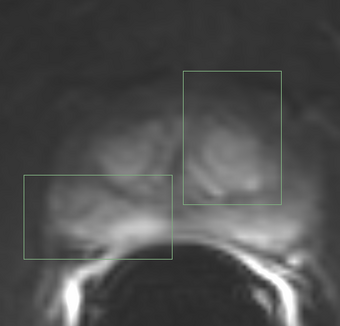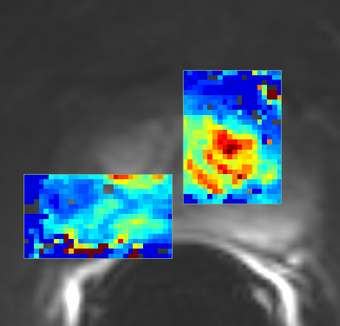# Difference between revisions of "Documentation/Nightly/Modules/PkModeling"

Home < Documentation < Nightly < Modules < PkModeling
 For the stable Slicer documentation, visit the 4.10 page.

# Introduction and Acknowledgements

Extension: PkModeling
Acknowledgments: This work is part of the National Alliance for Medical Image Computing (NA-MIC), funded by the National Institutes of Health through the NIH Roadmap for Medical Research, and by National Cancer Institute as part of the Quantitative Imaging Network initiative (U01CA151261) and QIICR (U24CA180918).
Implementation of the pharmacokinetics modeling was contributed by Yingxuan Zhu and Jim Miller from GE Research.
Author: Yingxuan Zhu (while at GE), Andrey Fedorov (SPL), John Evans (MGH), Jim Miller (GE)
Contact: Jim Miller, <email>millerjv@ge.com</email>GE Global ResearchNational Alliance for Medical Image Computing (NA-MIC)Quantitative Image Informatics for Cancer Research

# Module Description

 PkModeling (Pharmacokinetics Modeling) calculates quantitative parameters from Dynamic Contrast Enhanced DCE-MRI images. This module performs two operations: Converts signal intensities to concentration values. The concentration values are used to calculate quantitative parameters. Calculates quantitative parameters from concentration values. These parameters include: Ktrans Volume transfer constant between blood plasma and EES (extracellular-extravascular space) at each voxel (min^-1) Ve Fractional volume for extracellular space at each voxel MaxSlope Maximum slope in the time series curve of each voxel AUC Area under the curve of each voxel, measured from bolus arrival time to the end time of interval, normalized by the AUC of the AIF

# Use Cases

• estimation of quantitative perfusion parameters from DCE MRI
• treatment response evaluation
• breast, prostate, brain DCE MRI analysisSample frame from a breast DCE MRI dataset (one of the datasets presented in a study by Huang et al.Ktrans map result of PK modeling using population AIF (Parker et al., )Sample frame from a prostate DCE MRI dataset (QIN-PROSTATE-002 from TCIA QIN-PROSTATE collection)Ktrans map result of PK modeling using population AIF (Parker et al., )

• test

# Panels and their use

 PkModeling Parameters: T1 Blood Value (milliseconds) T1 Tissue Value (milliseconds) (default value is the published value for prostate tissue estimated in healthy individuals (see Ref. de Bazelaire et al.) r1 Relaxivity Value of the contrast agent, L x mol^(-1) x s^(-1). This value is contrast agent specific. Default setting of 0.0039 corresponds to Gd-DPTA (Magnevist) at 3T, see Ref. Pintaske et al. You will need to adjust this setting based on the magnet signal strength and contrast agent. Hematocrit Value. Volume percentage of red blood cells in blood. AUC Time Interval Value: Time interval for AUC calculation Use Population AIF: A mean AIF is calculated from a functional form instead of from the input using the aifMask or a prescribed AIF. See Ref. Parker et al. IO Input 4D Image: 4D DCE-MRI data T1 Map Image: T1 Map AIF Mask Image: Mask designating the location of the arterial input function (AIF). AIF can either be calculated from the input using the aifMask, prescribed directly in concentration units using the prescribedAIF option, or via a population AIF. Prescribed AIF: Prescribed arterial input function (AIF). AIF can either be calculated from the input using the aifMask option, via a population AIF, or can be prescribed directly in concentration units using the prescribedAIF option. An example of how a prescribed AIF can be defined is in File:AIF example.mcsv.zip (unzip before importing into Slicer!). Note that the x column corresponds to timestamps in seconds, and the y column is the contrast agent concentration (NOT image signal intensity). Output Ktrans image: volume transfer constant between blood plasma and extravascular extracellular space (EES) (min^-1) Output ve image: volume of EES per unit volume of tissue Output fpv image: (or v_p) blood plasma volume per unit of tissue Output maximum slope image: maximum slope of the signal intensity curve between any two consecutive timepoints Output AUC image: area under the curve in the first 90 seconds Advanced options: BAT Calculation Mode: PeakGradient (Default) or UseConstantBAT Constant BAT: Constant Bolus Arrival Time Index (frame number) Output R-squared goodness of fit image: each pixel will be initialized to a value between 0 and 1 characterizing the goodness of fit. Larger values correspond to a better fit (see R^2 measure description) Output Bolus Arrival Time Image: the bolus arrival time calculated at each pixel

The following acquisition parameters should be available in the NRRD header of the input data (if you are analyzing a DICOM time series, they will typically be extracted from the DICOM data):

• TR Value: Repetition time (milliseconds)
• TE Value: Echo time (milliseconds)
• FA Value: Flip angle (degrees)
• Timestamps for the dynamic series (in milliseconds)

Here is an example how this information is represented in the NRRD header:

```MultiVolume.DICOM.EchoTime:=2.93
MultiVolume.DICOM.FlipAngle:=10
MultiVolume.DICOM.RepetitionTime:=6.13
MultiVolume.FrameIdentifyingDICOMTagName:=AcquisitionTime
MultiVolume.FrameIdentifyingDICOMTagUnits:=ms
```

Auto generated documentation of the parameters

Parameters:

• PkModeling Parameters: Parameters used for PkModeling
• T1 Blood Value (T1PreBloodValue): T1 value for blood.
• T1 Tissue Value (T1PreTissueValue): T1 value for tissue.
• Relaxivity Value (RelaxivityValue): Contrast agent relaxivity value. Default value corresponds to Gd-DPTA (Magnevist) at 3T.
• F Tolerance Value (FTolerance): Tolerance on function value for establishing convergence.
• G Tolerance Value (GTolerance): Tolerance on gradient value for establishing convergence.
• X Tolerance Value (XTolerance): Tolerance on parameter values for establishing convergence.
• Epsilon Value (Epsilon): Epsilon value.
• Maximum number of iterations (MaxIter): Maximum number of iterations in optimizing the parameter estimation at each voxel.
• Hematocrit Value (Hematocrit): Hematocrit value, volume percentage of red blood cells in blood.
• AUC Time Interval Value (AUCTimeInterval): AUC Time Interval value.
• Compute fpv (3-parameter model) (ComputeFpv): Enable estimation of fractional plasma volume at each voxel.
• Use Population AIF (UsePopulationAIF): Use generic population AIF.
• IO: Input/output parameters
• Input 4D Image (InputFourDImageFileName): Input 4D Image (txyz)
• ROI Mask Image (ROIMaskFileName): (Optional) Mask designating the voxels (non-zero) where the model should be fit. If not specified, the model will be fit to all voxels in the image.
• T1 Map Image (T1MapFileName): (Optional) Volume defining T1 value for each voxel.
• AIF Mask Image (AIFMaskFileName): Mask designating the location of the arterial input function (AIF). AIF can be calculated from a generic population AIF, the input using the aifMask or can be prescribed directly in concentration units using the prescribedAIF option.
• Prescribed AIF (PrescribedAIFFileName): Prescribed arterial input function (AIF). AIF can either be calculated from the input using the aifMask option or can be prescribed directly in concentration units using the prescribedAIF option.
• Output Ktrans image (OutputKtransFileName): Output volume transfer constant between blood plasma and EES (extracellular-extravascular space) at each voxel.
• Output ve image (OutputVeFileName): Output fractional volume for extracellular space at each voxel.
• Output fpv image (OutputFpvFileName): Output fractional plasma volume at each voxel.
• Output maximum slope image (OutputMaxSlopeFileName): Output maximum slope in the time series curve of each voxel.
• Output AUC image (OutputAUCFileName): Output area under the curve (AUC) of each voxel, measured from bolus arrival time to the end time of interval, normalized by the AUC of the AIF.